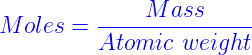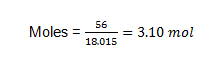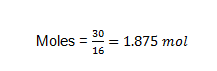# Grams to Moles Formula

The common or usual weight measurements in chemistry is figured out using moles. The conversion between moles and grams is typical. The grams to moles conversion is reliant on the atomic weight. One mole material comprises of Avogadro number of atoms. The grams to moles conversion formula is articulated as:We’ll examine some of the answered problems of gram to mole conversion in this segment.

SOLVED EXAMPLES

Problem 1: Convert 56g of water into moles?

Answer: We know that the mass of water is 56g. The molecular mass is noted for this calculation instead of atomic mass, as water is a molecule.

Molecular mass of water = 18.015g/mol

Grams to moles conversion equation is,Problem 2: Convert 30g of oxygen into corresponding moles?

Answer: We know that the mass of oxygen is 30g.

Atomic weight of oxygen = 16g/mol

Grams to Moles conversion equation is,